1.4 Polynomials  (Page 3/15)

 Page 3 / 15

Find the product.

$\left(3x+2\right)\left({x}^{3}-4{x}^{2}+7\right)$

$3{x}^{4}-10{x}^{3}-8{x}^{2}+21x+14$

Using foil to multiply binomials

A shortcut called FOIL is sometimes used to find the product of two binomials. It is called FOIL because we multiply the f irst terms, the o uter terms, the i nner terms, and then the l ast terms of each binomial.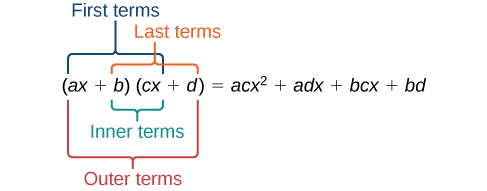The FOIL method arises out of the distributive property. We are simply multiplying each term of the first binomial by each term of the second binomial, and then combining like terms.

Given two binomials, use FOIL to simplify the expression.

1. Multiply the first terms of each binomial.
2. Multiply the outer terms of the binomials.
3. Multiply the inner terms of the binomials.
4. Multiply the last terms of each binomial.
6. Combine like terms and simplify.

Using foil to multiply binomials

Use FOIL to find the product.

$\left(2x-18\right)\left(3x+3\right)$

Find the product of the first terms.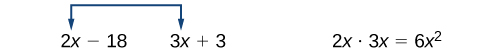Find the product of the outer terms.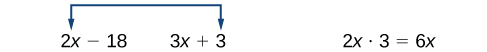Find the product of the inner terms.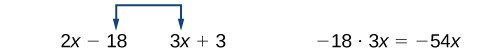Find the product of the last terms.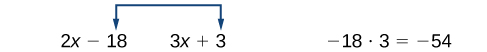Use FOIL to find the product.

$\left(x+7\right)\left(3x-5\right)$

$3{x}^{2}+16x-35$

Perfect square trinomials

Certain binomial products have special forms. When a binomial is squared, the result is called a perfect square trinomial    . We can find the square by multiplying the binomial by itself. However, there is a special form that each of these perfect square trinomials takes, and memorizing the form makes squaring binomials much easier and faster. Let’s look at a few perfect square trinomials to familiarize ourselves with the form.

Notice that the first term of each trinomial is the square of the first term of the binomial and, similarly, the last term of each trinomial is the square of the last term of the binomial. The middle term is double the product of the two terms. Lastly, we see that the first sign of the trinomial is the same as the sign of the binomial.

Perfect square trinomials

When a binomial is squared, the result is the first term squared added to double the product of both terms and the last term squared.

${\left(x+a\right)}^{2}=\left(x+a\right)\left(x+a\right)={x}^{2}+2ax+{a}^{2}$

Given a binomial, square it using the formula for perfect square trinomials.

1. Square the first term of the binomial.
2. Square the last term of the binomial.
3. For the middle term of the trinomial, double the product of the two terms.

Expanding perfect squares

Expand $\text{\hspace{0.17em}}{\left(3x-8\right)}^{2}.$

Begin by squaring the first term and the last term. For the middle term of the trinomial, double the product of the two terms.

${\left(3x\right)}^{2}-2\left(3x\right)\left(8\right)+{\left(-8\right)}^{2}$

Simplify

$\text{\hspace{0.17em}}9{x}^{2}-48x+64.$

Expand $\text{\hspace{0.17em}}{\left(4x-1\right)}^{2}.$

$16{x}^{2}-8x+1$

Difference of squares

Another special product is called the difference of squares    , which occurs when we multiply a binomial by another binomial with the same terms but the opposite sign. Let’s see what happens when we multiply $\text{\hspace{0.17em}}\left(x+1\right)\left(x-1\right)\text{\hspace{0.17em}}$ using the FOIL method.

$\begin{array}{ccc}\hfill \left(x+1\right)\left(x-1\right)& =& {x}^{2}-x+x-1\hfill \\ & =& {x}^{2}-1\hfill \end{array}$

The middle term drops out, resulting in a difference of squares. Just as we did with the perfect squares, let’s look at a few examples.

A laser rangefinder is locked on a comet approaching Earth. The distance g(x), in kilometers, of the comet after x days, for x in the interval 0 to 30 days, is given by g(x)=250,000csc(π30x). Graph g(x) on the interval [0, 35]. Evaluate g(5)  and interpret the information. What is the minimum distance between the comet and Earth? When does this occur? To which constant in the equation does this correspond? Find and discuss the meaning of any vertical asymptotes.
The sequence is {1,-1,1-1.....} has
how can we solve this problem
Sin(A+B) = sinBcosA+cosBsinA
Prove it
Eseka
Eseka
hi
Joel
June needs 45 gallons of punch. 2 different coolers. Bigger cooler is 5 times as large as smaller cooler. How many gallons in each cooler?
7.5 and 37.5
Nando
find the sum of 28th term of the AP 3+10+17+---------
I think you should say "28 terms" instead of "28th term"
Vedant
the 28th term is 175
Nando
192
Kenneth
if sequence sn is a such that sn>0 for all n and lim sn=0than prove that lim (s1 s2............ sn) ke hole power n =n
write down the polynomial function with root 1/3,2,-3 with solution
if A and B are subspaces of V prove that (A+B)/B=A/(A-B)
write down the value of each of the following in surd form a)cos(-65°) b)sin(-180°)c)tan(225°)d)tan(135°)
Prove that (sinA/1-cosA - 1-cosA/sinA) (cosA/1-sinA - 1-sinA/cosA) = 4
what is the answer to dividing negative index
In a triangle ABC prove that. (b+c)cosA+(c+a)cosB+(a+b)cisC=a+b+c.
give me the waec 2019 questionsByBy